JEE  >  Test: Evaluating Definite Integrals

# Test: Evaluating Definite Integrals

Test Description

## 10 Questions MCQ Test Mathematics (Maths) Class 12 | Test: Evaluating Definite Integrals

Test: Evaluating Definite Integrals for JEE 2023 is part of Mathematics (Maths) Class 12 preparation. The Test: Evaluating Definite Integrals questions and answers have been prepared according to the JEE exam syllabus.The Test: Evaluating Definite Integrals MCQs are made for JEE 2023 Exam. Find important definitions, questions, notes, meanings, examples, exercises, MCQs and online tests for Test: Evaluating Definite Integrals below.
Solutions of Test: Evaluating Definite Integrals questions in English are available as part of our Mathematics (Maths) Class 12 for JEE & Test: Evaluating Definite Integrals solutions in Hindi for Mathematics (Maths) Class 12 course. Download more important topics, notes, lectures and mock test series for JEE Exam by signing up for free. Attempt Test: Evaluating Definite Integrals | 10 questions in 10 minutes | Mock test for JEE preparation | Free important questions MCQ to study Mathematics (Maths) Class 12 for JEE Exam | Download free PDF with solutions
 1 Crore+ students have signed up on EduRev. Have you?
Test: Evaluating Definite Integrals - Question 1

### Evaluate: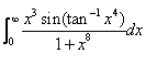Detailed Solution for Test: Evaluating Definite Integrals - Question 1

Let I = ∫(0 to pi/2)x3 sin(tan−1x4)/(1+x8)dx
Put tan−1 x4 = t
4x3/(1+x8)dx = dt
⇒x3dx/(1+x8) = dt/4
Now, I = 1/4∫sin t dt
=−[1/4 cos t]0 to pi/2 + c
= -1/4[cos(0) - cos(pi/2)] +c
= 1/4[-cos(pi/2) + cos(0)] +c
= ¼[-0+1]
= 1/4

Test: Evaluating Definite Integrals - Question 2

###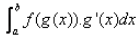Detailed Solution for Test: Evaluating Definite Integrals - Question 2

The given form of integral function (say ∫f(x)) can be transformed into another by changing the independent variable x to t,
Substituting x = g(t) in the function ∫f(x), we get;
dx/dt = g'(t)
or dx = g'(t).dt
Thus, I = ∫f(x).dx = f(g(t)).g'(t).dt
Therefore,  ∫f(g(x)).g'(x).dx  = f(b) - f(a)

Test: Evaluating Definite Integrals - Question 3

###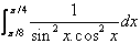Detailed Solution for Test: Evaluating Definite Integrals - Question 3

∫(π/8 to π/4)1/(sin2x cos2x)
= ∫(π/8 to π/4)(sin2x + cos^2x)/(sin2x cos2x) dx
= ∫(π/8 to π/4)(1/cos2x +1/sin2x) dx
= ∫(π/8 to π/4)(sec2 x dx + cosec2 x dx)
= [tan x - cot x](π/8 to π/4) + c
= [tan(π/8) - tan(π/4)] - [cot((π/8) - cot(π/4)]
= ((2)½ +1  - 1) - (1/(2)½ +1 - 1)
= 2

Test: Evaluating Definite Integrals - Question 4

The value of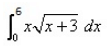is:

Test: Evaluating Definite Integrals - Question 5

If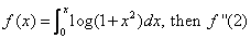is

Detailed Solution for Test: Evaluating Definite Integrals - Question 5

In the question, it should be f’(2) instead of f”(2)
Explanation:- f(x) = ∫(0 to x) log(1+x2)
f’(x) = 2xdx/(1+x2)
f’(2) = 2(2)/(1+(2)2)
= 4/5

Test: Evaluating Definite Integrals - Question 6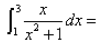Detailed Solution for Test: Evaluating Definite Integrals - Question 6

∫(1 to 3) x/(1+x2) dx
Put t = 1+x2
dt = 2x dx
= 1/2∫( 1 to 3) dt/t
= ½  log[t](1 to 3)
= ½[log(1+x2)](1 to 3)
= ½[log 10 - log 2]
= ½ (log(10/2)]
= ½[log 5]
= log(5)½

Test: Evaluating Definite Integrals - Question 7

If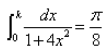then the value of k is:

Detailed Solution for Test: Evaluating Definite Integrals - Question 7

Let I=∫(0 to k) 1/[1 + 4x2]dx = π8
Now, ∫(0 to k) 1/[4(1/4 + x2)]dx
= 2/4[tan−1 2x]0 to k
= 1/2tan-1 2k − 0 = π/8
1/2tan−1 2k = π8
⇒ tan−1 2k = π/4
⇒ 2k = 1
∴ k = 1/2

Test: Evaluating Definite Integrals - Question 8

Evaluate: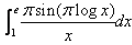Test: Evaluating Definite Integrals - Question 9

The value of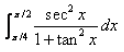is:​

Detailed Solution for Test: Evaluating Definite Integrals - Question 9

1 + tan2 x = sec2 x
∫(π/2 to π/4)(sec2 x)/(1 + tan2 x)
=> ∫(π/2 to π/4)(sec2 x)/(sec2 x) dx
= ∫1(π/2 to π/4) dx
= [x](π/2 to π/4)
= π/2 - π/4  =  π/4

Test: Evaluating Definite Integrals - Question 10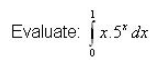Detailed Solution for Test: Evaluating Definite Integrals - Question 10

∫x(5x) dx
u = x,   dv = 5x dx
du = dx,   v = (5x/ln 5)
∫x(5x) dx = [x(5x)/ln 5](0 to 1) - ∫(0 to 1)(5x)/ln 5 dx
= 5/ ln 5 - 0 - 1/ln 5[5x/ln 5](0 to 1)
= 5/ln 5 - 5/(ln 5)2 + 1/(ln 5)2
=5/ln 5 - 4/(ln 5)2

## Mathematics (Maths) Class 12

209 videos|218 docs|139 tests
 Use Code STAYHOME200 and get INR 200 additional OFF Use Coupon Code
Information about Test: Evaluating Definite Integrals Page
In this test you can find the Exam questions for Test: Evaluating Definite Integrals solved & explained in the simplest way possible. Besides giving Questions and answers for Test: Evaluating Definite Integrals, EduRev gives you an ample number of Online tests for practice

## Mathematics (Maths) Class 12

209 videos|218 docs|139 tests